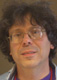# Hacking Primes in Mathematica

If this is too esoteric, skip it. I couldn’t figure out anywhere else to put it.

This morning, Tim Bray tweeted about a post on prime numbers and Benford’s law. To cut the esoterica short, one of the big problems in prime numbers is that people don’t know how they’re distributed. This post suggests that Benford’s Law describes the distribution of the first digit of prime numbers. One of the comments asked an important question: is this really just an artifact of base 10? Math really doesn’t “know anything” about bases, so if this idea doesn’t generalize to bases other than 10, it doesn’t mean much.

That challenge was a bit hard to ignore. A bit of futzing with Mathematica later, I got it. Here’s a graph of the distribution of prime numbers in base 36, for primes less than 36^5 (about 60 million, 3.5 million primes):
primes.tiff

Here’s the code (somewhat improved from the code that generated the graph)–only 5 very Lisp-like lines. For an explanation of the math, see the original article.

```With[{base = 36, ades = 5, a = 1.1}, nprimes := PrimePi[base^ades];